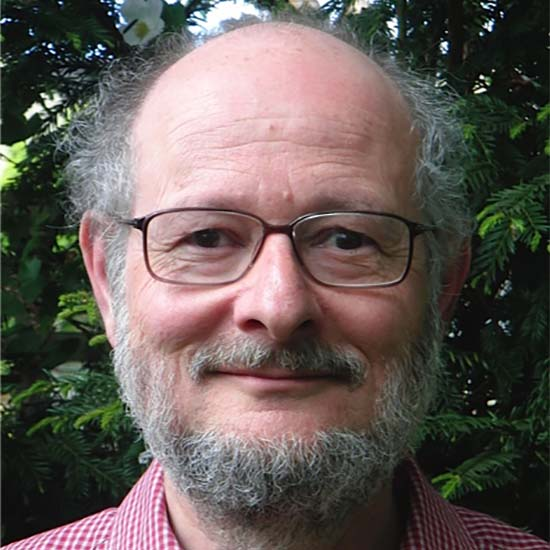# Nicholas MantonFellow

Elected: 1996

## Biography

Nicholas Manton is a Professor of Mathematical Physics, Department of Applied Mathematics and Theoretical Physics, University of Cambridge. He has made many seminal contributions to the theory of soliton-like particles in two and three dimensions. Solitons are localised, twisted structures in a field that are topologically stable. He calculated the forces between static and moving monopoles and vortices in gauge theories, leading to the geometrical idea of ‘moduli space’ dynamics. This has been applied to the classical, quantum and statistical mechanics of solitons. He has also developed the theory of skyrmions as a soliton model of atomic nuclei.

He discovered the unstable ‘sphaleron’ solution in the electroweak sector of the Standard Model of particle physics. The Higgs field is topologically twisted within a sphaleron. The sphaleron defines an energy scale for baryon and lepton number violation in the early universe — an energy scale within the range of the Large Hadron Collider. Nicholas’s other work includes the construction of a 10-dimensional theory containing supergravity and Yang–Mills theory, which is a low-energy limit of superstring theory.

## Professional positions

Professor of Mathematical Physics, Department of Applied Mathematics and Theoretical Physics, University of Cambridge

## Interest and expertise

### Subject groups

• Mathematics
• Applied mathematics and theoretical physics
• Astronomy and physics
• Elementary particle physics

### Keywords

Particle Physics, Atomic nuclei, Topological solitons , Skyrmions